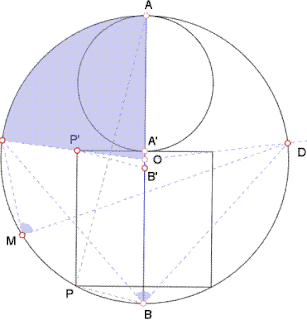## Wednesday, March 24, 2010

### Fox 265 - Solutions

Joe has already posted a straightforward solution for Fox 265. Below are several more:

Pure Geometry - Little Trigonometry by Bleaug:a=angle(CMD)=angle(CBD)=angle(AOC)
PP'=AA'=2.A'P' therefore tan(angle(PAB))=1/4. Take B' such that PB//P'B' then angle(A'P'B')=angle(PAB). By construction OA'=OB', so tan(a)=A'P'/OA'=2.A'P'/A'B'=2/tan(angle(A'P'B'))=8.

More Trigonometry by Yu:Radius of the small circle is r, radius of the large circle is R.
tanθ = 1/4, tanΦ=tan2θ=2tanθ/(1-tanθ^2) = 8/15
so, r / sqrt(R^2 - r^2) = 8/15 => R = (17/8)r
tan* = r / (R - 2r) = 8.

Nice use of Pythagoras By Bob Ryden:Inscribed m(ABC) = (1/2) m(AOC) = m(POQ), so we can find m(POQ).
Let M be the midpoint of LN. Draw OM and ON.
Let radius of small circle = a.
Let PO = x.
Then radius of large circle = 2a + x.
OM = 2a – x.
MN = a.
ON = radius of large circle = 2a + x.
OM^2 + MN^2 = ON^2.
(2a – x)^2 + a^2 = (2a + x)^2
a = 8x
tan (ABC) = tan (POQ) = a/x = 8.

http://www.8foxes.com/